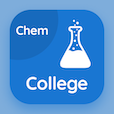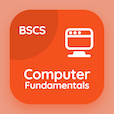Colleges Online Courses

College Chemistry Quizzes

College Chemistry Quiz PDF - Complete

# Quantum Theory Quiz MCQ Online p. 33

Practice Quantum Theory quiz questions and answers PDF, quantum theory trivia questions 33 to learn online College Chemistry course for online classes. Atomic Structure MCQ questions, quantum theory Multiple Choice Questions (MCQ) for online college degrees. "Quantum Theory Quiz" PDF eBook: van der waals equation, hydrogen spectrum, basic chemistry, properties of crystalline solids, quantum theory test prep for online degrees.

"The distance between two adjacent crests or troughs is called" MCQ PDF: frequency, wave number, wave length, and radiation for online degrees. Solve atomic structure questions and answers to improve problem solving skills for online degrees.

## Trivia Quiz on Quantum Theory MCQs

MCQ: The distance between two adjacent crests or troughs is called

wave number
frequency
wave length

MCQ: The forms which show polymorphism is called

polymorphs
isomorphs
parallel structures
cubic crystals

MCQ: Ascorbic acid is another name for vitamin

A
B
C
D

MCQ: Balmer series was discovered in

1867
1887
1789
1901

MCQ: The gas chlorine has a high value of constant

b
a
c
d

### More Quizzes from College Chemistry Course